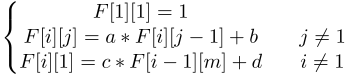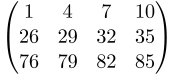## Day 2, Problem 1 - Matrix Game

TingTing is a girl that loves matrices. One day, she wants to use a computer to generate a giant n row by m column matrix (you don't have to worry about how she'll store it). Her generated matrix will satisfy a mystical property: if we use F[i][j] to represent the cell in the i-th row and j-th column, then F[i][j] will satisfy the following system of equations:$\displaystyle \left\{\begin{matrix} F = 1 \\ F[i][j] = a*F[i][j-1]+b && j \neq 1 \\ F[i] = c*F[i-1][m]+d && i \neq 1 \end{matrix}\right.$

where a, b, c, and d are given constants.

TingTing would like to know the value of F[n][m] and she would like you to help her. Since the final value may be very large, you are only required to output it modulo 1,000,000,007.

### Input Format

The input will contain the six integers n, m, a, b, c, and d.

### Output Format

Output a single integer, the value of F[i][j] modulo 1,000,000,007.

### Sample Input

3 4 1 3 2 6


### Sample Output

85


### Explanation

The matrix in the example is:$\textstyle \begin{pmatrix} 1 & 4 & 7 & 10 \\ 26 & 29 & 32 & 35 \\ 76 & 79 & 82 & 85 \end{pmatrix}$

### Constraints

Test CaseConstraints
11 ≤ n, m ≤ 10; 1 ≤ a, b, c, d ≤ 1000
21 ≤ n, m ≤ 100; 1 ≤ a, b, c, d ≤ 1000
31 ≤ n, m ≤ 103; 1 ≤ a, b, c, d ≤ 109
4
51 ≤ n, m ≤ 109; 1 ≤ a = c ≤ 109; 1 ≤ b = d ≤ 109
61 ≤ n, m ≤ 109; a = c = 1; 1 ≤ b, d ≤ 109
71 ≤ n, m, a, b, c, d ≤ 109
8
9
10
111 ≤ n, m ≤ 101,000; a = c = 1; 1 ≤ b, d ≤ 109
121 ≤ n, m ≤ 101,000; 1 ≤ a = c ≤ 109; 1 ≤ b = d ≤ 109
131 ≤ n, m ≤ 101,000; 1 ≤ a, b, c, d ≤ 109
14
151 ≤ n, m ≤ 1020,000; 1 ≤ a, b, c, d ≤ 109
16
171 ≤ n, m ≤ 101,000,000; a = c = 1; 1 ≤ b, d ≤ 109
181 ≤ n, m ≤ 101,000,000; 1 ≤ a = c ≤ 109; 1 ≤ b = d ≤ 109
191 ≤ n, m ≤ 101,000,000; 1 ≤ a, b, c, d ≤ 109
20

Point Value: 20 (partial)
Time Limit: 1.00s
Memory Limit: 256M